Anzeige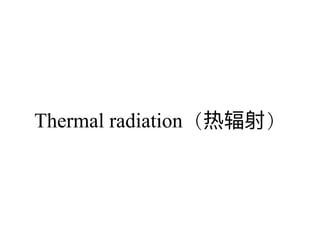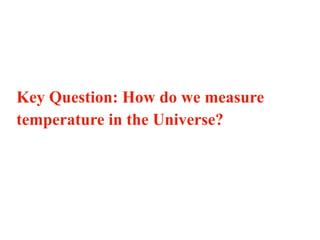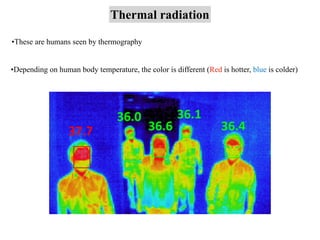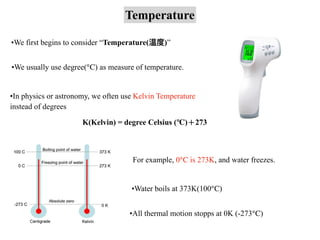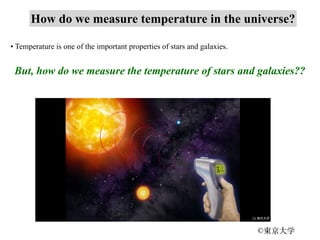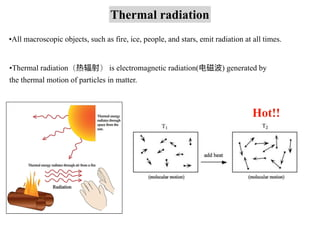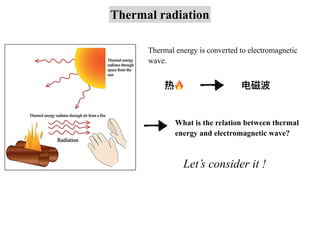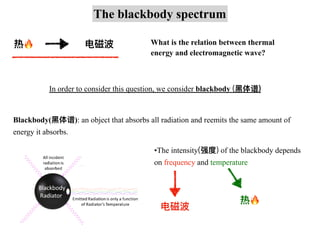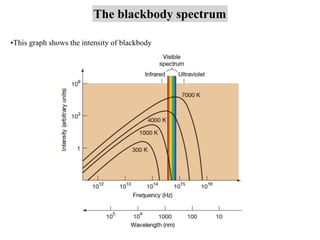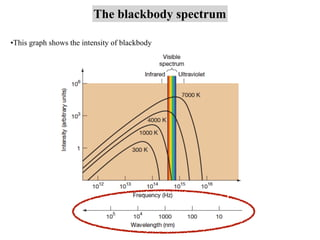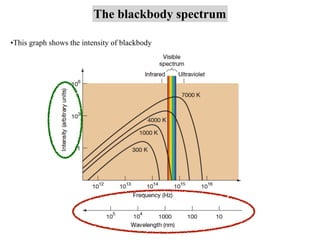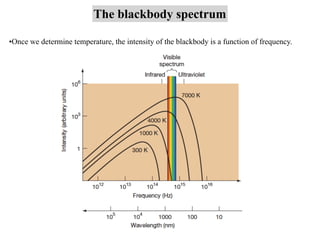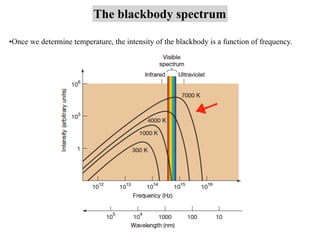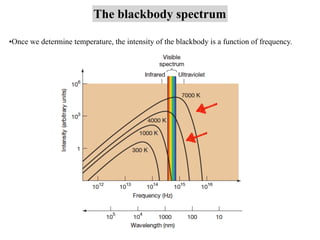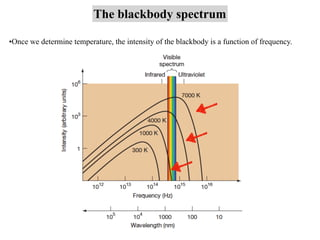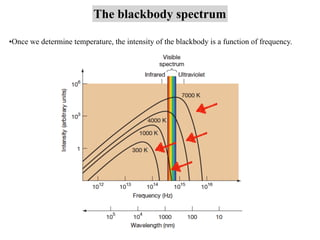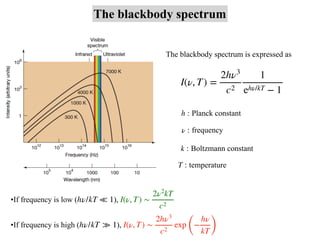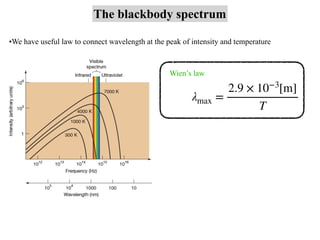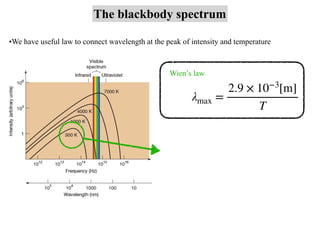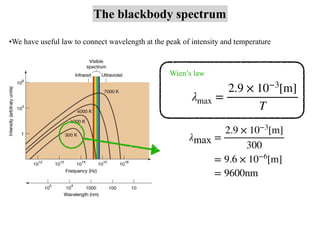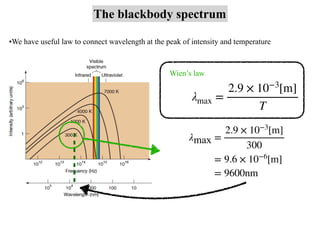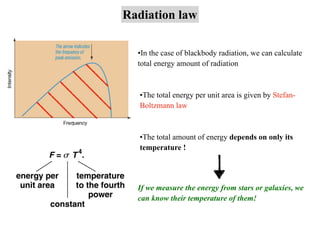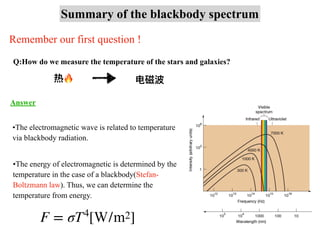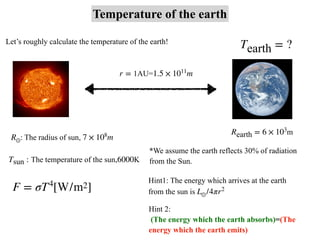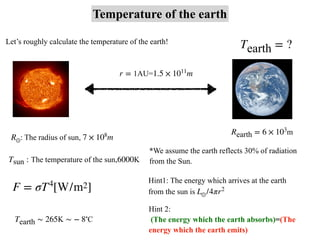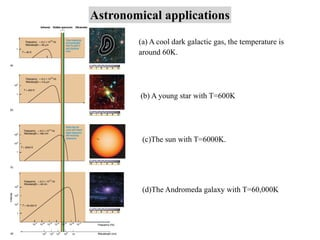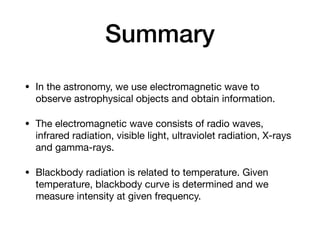1 von 29
Anzeige

### lecture7

1. Introduction to modern astronomy 7 島袋隼⼠(Hayato Shimabukuro)（云南⼤学、 SWIFAR） ©GETTYIMAGES
3. Key Question: How do we measure temperature in the Universe?
4. Do you feel hot??
5. Thermal radiation •These are humans seen by thermography •Depending on human body temperature, the color is different (Red is hotter, blue is colder)
6. Temperature •We first begins to consider “Temperature(温度)” •We usually use degree(℃) as measure of temperature. •In physics or astronomy, we often use Kelvin Temperature instead of degrees K(Kelvin) = degree Celsius (℃)＋273 For example, 0℃ is 273K, and water freezes. •All thermal motion stopps at 0K (-273℃) •Water boils at 373K(100℃)
7. How do we measure temperature in the universe? • Temperature is one of the important properties of stars and galaxies. But, how do we measure the temperature of stars and galaxies?? ©東京⼤学
8. Thermal radiation •All macroscopic objects, such as fire, ice, people, and stars, emit radiation at all times. •Thermal radiation（热辐射） is electromagnetic radiation(电磁波) generated by the thermal motion of particles in matter. Hot!!
9. Thermal radiation Thermal energy is converted to electromagnetic wave. What is the relation between thermal energy and electromagnetic wave? Let’s consider it ! 热🔥 电磁波
10. The blackbody spectrum 热🔥 电磁波 What is the relation between thermal energy and electromagnetic wave? Blackbody(⿊体谱): an object that absorbs all radiation and reemits the same amount of energy it absorbs. In order to consider this question, we consider blackbody (⿊体谱) •The intensity(强度) of the blackbody depends on frequency and temperature 热🔥 电磁波
11. The blackbody spectrum •This graph shows the intensity of blackbody
12. The blackbody spectrum •This graph shows the intensity of blackbody
13. The blackbody spectrum •This graph shows the intensity of blackbody
14. The blackbody spectrum •Once we determine temperature, the intensity of the blackbody is a function of frequency.
15. The blackbody spectrum •Once we determine temperature, the intensity of the blackbody is a function of frequency.
16. The blackbody spectrum •Once we determine temperature, the intensity of the blackbody is a function of frequency.
17. The blackbody spectrum •Once we determine temperature, the intensity of the blackbody is a function of frequency.
18. The blackbody spectrum •Once we determine temperature, the intensity of the blackbody is a function of frequency.
19. The blackbody spectrum I(ν, T) = 2hν3 c2 1 ehν/kT − 1 The blackbody spectrum is expressed as h : Planck constant ν : frequency T : temperature •If frequency is low ( ), hν/kT ≪ 1 I(ν, T) ∼ 2ν2 kT c2 k : Boltzmann constant •If frequency is high ( ), hν/kT ≫ 1 I(ν, T) ∼ 2hν3 c2 exp ( − hν kT )
20. •We have useful law to connect wavelength at the peak of intensity and temperature The blackbody spectrum λmax = 2.9 × 10−3 [m] T Wien’s law
21. •We have useful law to connect wavelength at the peak of intensity and temperature The blackbody spectrum λmax = 2.9 × 10−3 [m] T Wien’s law
22. •We have useful law to connect wavelength at the peak of intensity and temperature The blackbody spectrum λmax = 2.9 × 10−3 [m] T Wien’s law λmax = 2.9 × 10−3 [m] 300 = 9.6 × 10−6 [m] = 9600nm
23. •We have useful law to connect wavelength at the peak of intensity and temperature The blackbody spectrum λmax = 2.9 × 10−3 [m] T Wien’s law λmax = 2.9 × 10−3 [m] 300 = 9.6 × 10−6 [m] = 9600nm
24. Radiation law •The total energy per unit area is given by Stefan- Boltzmann law •The total amount of energy depends on only its temperature ! •In the case of blackbody radiation, we can calculate total energy amount of radiation If we measure the energy from stars or galaxies, we can know their temperature of them!
25. Summary of the blackbody spectrum Q:How do we measure the temperature of the stars and galaxies? Remember our first question ! Answer •The electromagnetic wave is related to temperature via blackbody radiation. 热🔥 电磁波 •The energy of electromagnetic is determined by the temperature in the case of a blackbody(Stefan- Boltzmann law). Thus, we can determine the temperature from energy. F = σT4 [W/m2]
26. Temperature of the earth F = σT4 [W/m2] Let’s roughly calculate the temperature of the earth! : The radius of sun, R⊙ 7 × 108 m 1AU= r = 1.5 × 1011 m Tsun : The temperature of the sun,6000K Rearth = 6 × 103 m Hint 2: (The energy which the earth absorbs)=(The energy which the earth emits) *We assume the earth reflects 30% of radiation from the Sun. Hint1: The energy which arrives at the earth from the sun is L⊙/4πr2 Tearth = ?
27. Temperature of the earth F = σT4 [W/m2] Let’s roughly calculate the temperature of the earth! : The radius of sun, R⊙ 7 × 108 m 1AU= r = 1.5 × 1011 m Tsun : The temperature of the sun,6000K Rearth = 6 × 103 m Hint 2: (The energy which the earth absorbs)=(The energy which the earth emits) *We assume the earth reflects 30% of radiation from the Sun. Hint1: The energy which arrives at the earth from the sun is L⊙/4πr2 Tearth ∼ 265K ∼ − 8∘ C Tearth = ?
28. Astronomical applications (a) A cool dark galactic gas, the temperature is around 60K. (b) A young star with T=600K (c)The sun with T=6000K. (d)The Andromeda galaxy with T=60,000K
29. Summary • In the astronomy, we use electromagnetic wave to observe astrophysical objects and obtain information. • The electromagnetic wave consists of radio waves, infrared radiation, visible light, ultraviolet radiation, X-rays and gamma-rays. • Blackbody radiation is related to temperature. Given temperature, blackbody curve is determined and we measure intensity at given frequency.
Anzeige